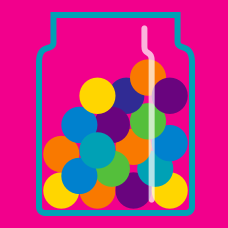Probability

# Two-Events Conditionals

A survey on the preference for great composers in the 20$^\text{th}$ century was conducted to 100 music critics. A part of the result is shown in the table below. What is the probability that a randomly chosen critic likes Stravinsky?

$\begin{array}{lcc} & \text{like Schoenberg} & \text{hate Schoenberg} \\ \text{like Stravinsky} & 40 & 25 \\ \text{hate Stravinsky} & 20 & 15 \end{array}$

Xander flips a coin and tosses a 6-sided dice. What is the probability that either the coin lands on Heads, or the dice lands on 4?

100 high school students are asked if they speak French. 70% of male students answer that they do not speak French, while 40% of female students answer they speak French. It is known that 40% of the responding students are female. If a randomly chosen student answers that he/she speaks French, what is the probability that this student is a female?

In a school, $30\%$ of the students are female, and $50\%$ of the students walk to school. If $20\%$ of the students are female and walk to school, what is the probability that a randomly chosen student is female or walks to school?

A card is drawn randomly from a standard deck of cards. What is the probability that the card drawn is a 2 or a Spade?

Details and Assumptions:

A standard deck of cards consists of 52 cards, formed by 13 ranks (Ace, 2, 3, 4, 5, 6, 7, 8, 9, 10, Jack, Queen and King) of 4 suits (clubs, diamonds, hearts and spades).

×